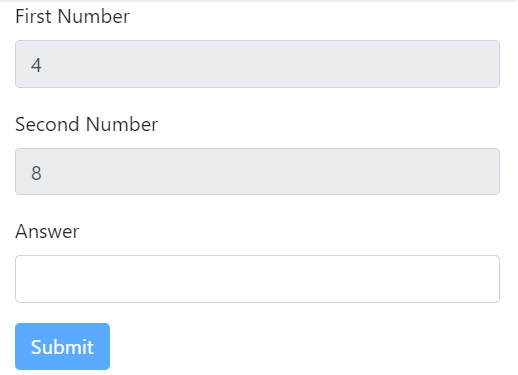# How to create a math Captcha using Angular 2

Angular is a common framework for developing front end application using typescript. Angular provides a lot of features out of the box. Sometimes we need some features which are not available in the framework like comparing two form control.
As usual, I like to define some goals so that the solution does make sense to you. What I need is:
To create a math captcha (Not used for production) where the user will be presented with some Random maths question (in our case sum of two random number 😊😊) and when user enter the correct value submit button will be enabled.Let’s start the tutorial.
create an angular application by executing the following command
``````ng new app fgValidationDemo
``````
Import `ReactiveFormsModule` from `@angular/form` as shown below
``````import { NgModule } from "@angular/core";
import { BrowserModule } from "@angular/platform-browser";
import { FormsModule, ReactiveFormsModule } from "@angular/forms";

import { AppComponent } from "./app.component";

@NgModule({
imports: [BrowserModule, FormsModule, ReactiveFormsModule],
declarations: [AppComponent],
bootstrap: [AppComponent]
})
export class AppModule {}

``````
open `app.component.ts` and create a FormGroup with three form control named `firstNnumber`,`secondNumber` and `answer` as shown below and then call the function inside constructor of the component.
``````initForm() {
this.mathFormGroup = new FormGroup(
{
firstNumber: new FormControl(this.randomNumber(), [
Validators.required
]),
secondNumber: new FormControl(this.randomNumber(), [
Validators.required
]),
});
}
``````
Open app.component.html and bind these controls with HTML
``````<div class="container">
<div class="row">
<div class="col-md-6">
<form [formGroup]="mathFormGroup">
<div class="form-group">
<label for="firstNumber">First Number</label>
<input type="number" class="form-control"
formControlName="firstNumber"
disabled="true"
id="firstNumber">

</div>
<div class="form-group">
<label for="secondNumber">Second Number</label>
<input type="number" class="form-control"
disabled="true"

formControlName="secondNumber"
id="secondNumber" >

</div>
<div class="form-group">
<input type="number" class="form-control"

</div>

<button type="submit" [disabled]="!mathFormGroup.valid"class="btn btn-primary">Submit</button>
</form>
</div>
</div>
<pre>
{{mathFormGroup.value|json}}
{{mathFormGroup.valid|json}}
</pre>
</div>
``````
This html is self explanorty
*Now it’s time to add the validation. If you see the signature of the `FormGroup` API, which takes the second argument `validatorOrOpts`. we will modify the `initForm` function and call the function `answerValidator.`
``````class FormGroup extends AbstractControl {
constructor(controls: { [key: string]: AbstractControl; },  validatorOrOpts?: ValidatorFn )
``````
``````initForm() {
this.mathFormGroup = new FormGroup(
{
firstNumber: new FormControl(this.randomNumber(), [
Validators.required
]),
secondNumber: new FormControl(this.randomNumber(), [
Validators.required
]),
},
);
}
console.log(form.value);
const { firstNumber, secondNumber, answer } = form.value;
if (+answer === parseInt(firstNumber) + parseInt(secondNumber)) {
return null;
}
return { math: true };
}

``````
This idea is straightforward, In `answerValidator` function we have access to `FormGroup`. We get the value of the form control and compare their value and return the error based on the condition in our case answer control value should be equal to the sum of the first and second number.
The same technique can be used in comparing the password.
DEMO